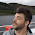## Tuesday, September 18, 2012

### A Case for Tuples

One of the more exciting new features in C++11 is the std::tuple template. A tuple is a struct pretending to be an array; the variable names are replaced with anonymous tuple indices. By replacing identifiers with more abstract indices, we can create a more abstract object. For example, cartesian coordinates (x, y) and complex coordinates (phase, magnitude) could both be represented by tuple<float, float>. Unlike an array, the elements can have different types.

Consider any C-style struct. The struct's member variables can be mapped directly to tuple elements. The first variable and index zero could be paired, followed by the second variable and index one. This would continue until the last member variable was paired with index N, where N is one less than the number of variables in the struct. With this mapping, it just so happens that the tuple’s elements are in the same order as the struct’s records, though this need not be the case. Regardless of the chosen mapping, a tuple abstracts away the names of the members, so instead of “position” and “velocity”, we have element one and element two.

To demonstrate a tuple’s abstraction potential, here is a simple example:

```struct Move {
float velocity_;
float position_;

Move(float pos, float vel)
: velocity_ (vel)
, position_ (pos) {
}

inline update(float dt) {
position_ += velocity_ * dt;
}
};
```
Let’s reimplement this class using a tuple instead of member variables:

```template<typename T>
struct Integrate {
std::tuple<T, T> tuple_;

Integrate(T s, T ds)
: tuple_ (ds, s) {
}

inline update(float dt) {
std::get<1>(tuple_) += std::get<0>(tuple_) * dt;
}
};
```
We’ve successfully abstracted away all of the context about “moving”. Now this implementation can be used for other tasks such as calculating throughput for a network interface. Without this context, unfortunately, the abstraction is more difficult to use. A name is obviously better than an index, so it seems as if we’ve taken a step back. Lets instead focus on what we get by abstracting away this context. What if we wish to add another “order” to the integration? For example, we are developing a game and we want to allow acceleration of a game entity due to gravity. We can add another element to our tuple:

```template<typename T>
struct Integration2 {
std::tuple<T, T, T> tuple_;

Integration(T s, T ds, T ds2)
: tuple_ (ds2, ds, s) {
}

inline update(float dt) {
std::get<1>(tuple_) += std::get<0>(tuple_) * dt;
std::get<2>(tuple_) += std::get<1>(tuple_) * dt;
}
};
```
Without the context, it is easy to see that this is a pattern that can be generalized. Why have two different definitions of this class when instead we could have a single template that can define any number of iterated integrals?
```// "Recursive" Case
template <int N>
struct UpdateTupleImpl {
template <typename...T>
static void update(std::tuple<T...>& tuple, float dt) {
UpdateTupleImpl<N-1>::template update<T...>(tuple, dt);
std::get<N+1>(tuple) += std::get<N>(tuple) * dt;
}
};

// Terminal Case
template <>
struct UpdateTupleImpl<1> {
template <typename...T>
static void update(std::tuple<T...>& tuple, float dt) {
std::get<1>(tuple) += std::get<0>(tuple) * dt;
}
};

// Wrap it up in a helper - deduce template parameters
template <typename...T>
inline void integrate(std::tuple<T...>& tuple, float dt) {
UpdateTupleImpl<sizeof...(T)-1>::template update<T...>(tuple, dt);
}

// Example
std::tuple<float, float, float> vel(1.0f, 5.0f, 10.0f);
integrate(vel, 100.0f);
integrate(vel, 100.0f);
float position = std::get<1>(vel);
```
Along with demonstrating C++’s ability to define powerful abstractions, this example also shows how ugly and verbose the language can be. Our template parameters can be any mix of types (we can have floats, doubles and ints mixed for example), but all the types must support the += operator and float multiplication. This example isn't supposed to be useful or used in practice; it is just a simple demonstration of abstraction using tuples.

In the example above, the “recursive” case isn’t actually recursive. Because it is the template parameter that varies, it is actually calling a completely different function with each iteration. We also know the number of iterations at compile-time, so the functions are inlined and the implementation is as efficient as the more concrete example.

We’ve already covered one of the major disadvantages of a tuple: the loss of context caused by abstracting away identifiers. Not only do we have to know what a tuple’s elements’ values represent whenever we use it, we must also know the values’ types and their positions within the tuple. Something very interesting happens if every element of the tuple has a different type. It becomes possible to unambiguously address a tuple element by its type alone, thus abstracting both identifiers and tuple element positions away. Its even possible for the type names to contain enough context to make up for losing the identifiers.

```typedef Integration<Velocity, Position> Mover;
// initial velocity, and initial position
Mover mv({0.0f}, {100.0f});
update(mv, 100.0f);
update(mv, 100.0f);
float position = getType<Position>(mv.tuple_);
```
Unfortunately std::tuple does not have a uniqueness constraint. Even if it did, we’d have to define the unique types for the tuple. The next article in this series will address these issues with an alternative to std::tuple.

#### 4 comments:

1.Is it more correct to describe complex coordinates as (real, imaginary) than (phase, magnitude)?

1.Yes... yes it is. I've been polluted by my EE background...

2.struct Move {
Integration(float pos, float vel)

I may be wrong, but constructor names look pretty messed up.

1.You are not wrong at all! Fixed.